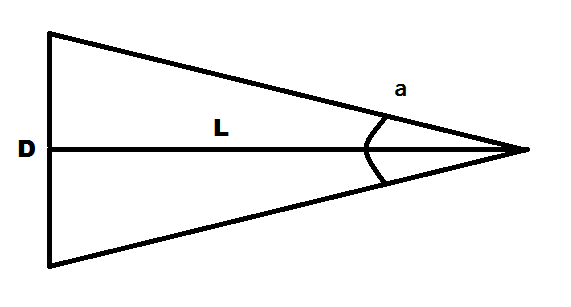homechevron_rightStudychevron_rightMathchevron_rightGeometry

# Angular size, linear size and distance

These calculators computes value of angular size or linear size or distance from the other two'sThis content is licensed under Creative Commons Attribution/Share-Alike License 3.0 (Unported). That means you may freely redistribute or modify this content under the same license conditions and must attribute the original author by placing a hyperlink from your site to this work https://planetcalc.com/1897/. Also, please do not modify any references to the original work (if any) contained in this content.The angular size (angular diameter, apparent size) of an object as seen from a given point is the visual diameter of the object measured as an angle. The visual diameter is the diameter of the perspective projection of the object on a plane through its center that is perpendicular to the viewing direction. Look at the picture.

The angular size, linear size and distance can be calculated using the formulas:

Thus, we can find out distance from the object if we knew its size and its angular size. Binoculars often have special marks which helps to find out angular size of observed object.

Also we can find out object size from its angular size and distance from it. And of course its angular size from its linear size and distance. This is a very frequent way of measuring things in astronomy. In astronomy the sizes of objects in the sky are often given in terms of their angular diameter as seen from Earth, rather than their actual sizes.

Below the calculators which can compute any parameter from two others. By default, distance from sun to earth, sun's diameter and sun's average angular size are used.#### Distance from angular size and linear size

°
Digits after the decimal point: 2
Distance#### Linear size from angular size and distance

°
Digits after the decimal point: 2
Linear size#### Angular size from distance and linear size

Angular sizePLANETCALC, Angular size, linear size and distance